# CBSE Class 9 Mathematics Circles VBQs

CBSE Class 9 Mathematics Circles VBQs read and download in pdf. Value Based Questions come in exams for Circle in Standard 9 and are easy to learn and helpful in scoring good marks. You can refer to more chapter wise VBQs for Class 9 Circle and also get latest topic wise very useful study material as per latest 2021 NCERT book for Class 12 Circle and all other subjects for free on Studiestoday designed as per latest Grade 9 CBSE, NCERT and KVS syllabus and examination pattern

CIRCLES

Question. Three friends ordered 3 pizzas of same size for them. Just then twomore friends joined them. They decided to share 3 pizzas among all 5 of them.
(a) Find the area of the share of pizza each child gets if the radius ofeach pizza is 7 cm.
(b) Which values of children are depicted here ?
(a) 92.4 cm2  (b) co-operatio

Question. During ‘Van Mahotsav’ celebration in a school a circular plot in the corner of the school was chosen. Five groups of students were selected to plant trees in each part as shown in the fig.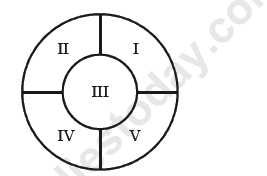(a) Which group gets the maximum area to develop and by how much, if the two radii are 210 cm & 105 cm respectively.
(b) What values can be inculcated in students through this activity ?
(a) Group III, 8662.5 sq. cm  (b) Environmental protection, beauty

Question. The Indian hockey federation organized a friendly hockey match between India and Pakistan on a circular ground. The sale proceed of this match shall be donated to an orphanage. A rectangular turf is spread on the ground as shown in the figure.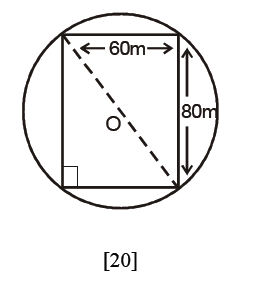(b) Which social values is depicted here ?
(c) How does donation to charitable organisations help in the development of society ?
(a) 50 m   (b) Helpfulness, co-operation
(c) This money is utilized for welfare of orphans.

Question. A school decided to paint its inner boundary wall. They planned to write quotations and social messages for the children on the circularareas of radius 40 cm each on the wall along a straight line.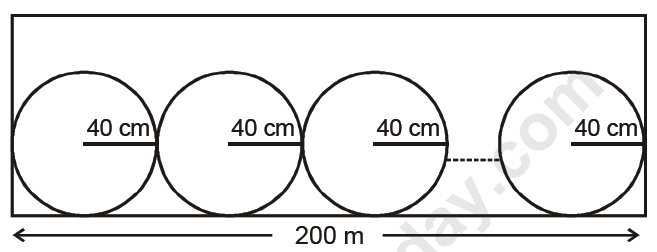If the boundary wall is 200 m long
(a) How many circles can be drawn ?
(b) What values are inculcated in the students by this activity ?
(a) 250  (b) Beauty

Question. A group of 66 students went for a picnic. They halted at a park for their lunch. They made a big circle and each child was 50 cm away from the other child along the circle.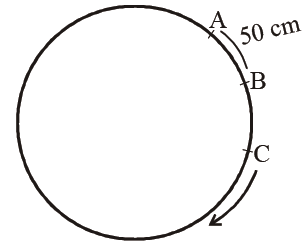(a) Find the radius of the circle they formed.
(b) What values do these students possess ?
(a) 5.25 m     (b) Co-operation, happiness

Question. An amusement fair was organised in a circular park for the children of slum clusters. Free food was supplied to them at 4 stalls situated at A, B, C and D as shown in the figure.(a) Find the distance between any two adjacent food stalls if theradius of the park is 30 m.
(b) Are such activities helpful for society ? What are the other social issues for which such compaigns are required ?
(a) 30√ 2 m   (b) Yes, for the inmates of old age home, orphanages, handicapped children etc.

Question. Children of a colony decided to assist and help an old couple of the colony in developing their vacant plot as flower bed as shown here. The,radii of the two circles is 245 cm and 210 cm.
(a) Find the area of the grass cover.
(b) Which values are depicted by the children?
(a) 50050 sq. cm (b) Helpfulness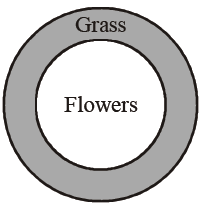Question. 3 STD booths situated at A, B and C in the fig. are operated by handicapped persons. These three booths are equidistant from each other as shown in the figure.(a) Find angle BOC
(b) Do you think employment provided to handicapped persons is important for the development of a society ? Justify your answer.
(a) 120°  (b) Yes

Question. During a practical activity in maths lab, students were using circular Geo board. The angle subtended by an arc at the centre is (2a+50°) Sakshi calculated angle BAC as (a+25°)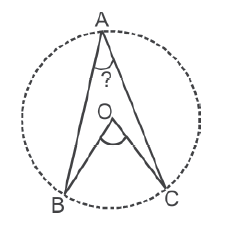(a) Is her finding correct ? Justify it.
(b) Find angle BAC if a = 30
(c) Which values are depicted here ?
(a) Yes   (b) 55° (c) T r u t h ,scientific temper

Question. In a circle with centre O, ABCD is a cyclic quadrilateral.x+y = 90° depicts which of these values ?
(a) Social value (b) Freedom

angle BAD + angle BCD = 180° (opposites angles of a cyclic quadrilateral)

y+x+90 = 180

x + y = 90°
Truthfulness, knowledge

 CBSE Class 9 Mathematics Circles VBQs
 CBSE Class 9 Mathematics Construction VBQs
 CBSE Class 9 Mathematics Surface Areas And Volume VBQs
 CBSE Class 9 Mathematics Statistics VBQs
 CBSE Class 9 Mathematics Probability VBQs
 CBSE Class 9 Mathematics Linear Equations VBQs
 CBSE Class 9 Mathematics Quadrilaterals VBQs
 CBSE Class 9 Mathematics Areas Of Parallelograms And Triangles VBQs
 CBSE Class 9 Mathematics Value Based Questions